# 8.10. Binary Heap Implementation¶

## 8.10.1. The Structure Property¶

In order to make our heap work efficiently, we will take advantage of the logarithmic nature of the binary tree to represent our heap. In order to guarantee logarithmic performance, we must keep our tree balanced. A balanced binary tree has roughly the same number of nodes in the left and right subtrees of the root. In our heap implementation we keep the tree balanced by creating a complete binary tree. A complete binary tree is a tree in which each level has all of its nodes. The exception to this is the bottom level of the tree, which we fill in from left to right. Figure 1 shows an example of a complete binary tree.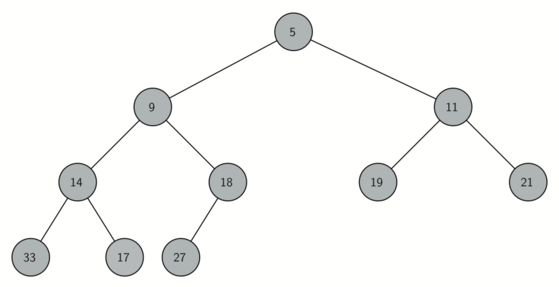Figure 1: A Complete Binary Tree¶

Another interesting property of a complete tree is that we can represent it using a single vector. We do not need to use nodes and references or even vectors of vectors. Because the tree is complete, the left child of a parent (at position $$p$$) is the node that is found in position $$2p$$ in the vector. Similarly, the right child of the parent is at position $$2p + 1$$ in the vector. To find the parent of any node in the tree, we can simply use Python’s integer division. Given that a node is at position $$n$$ in the vector, the parent is at position $$n/2$$. Figure 2 shows a complete binary tree and also gives the vector representation of the tree. Note the $$2p$$ and $$2p+1$$ relationship between parent and children. The vector representation of the tree, along with the full structure property, allows us to efficiently traverse a complete binary tree using only a few simple mathematical operations. We will see that this also leads to an efficient implementation of our binary heap.

## 8.10.2. The Heap Order Property¶

The method that we will use to store items in a heap relies on maintaining the heap order property. The heap order property is as follows: In a heap, for every node $$x$$ with parent $$p$$, the key in $$p$$ is smaller than or equal to the key in $$x$$. Figure 2 also illustrates a complete binary tree that has the heap order property.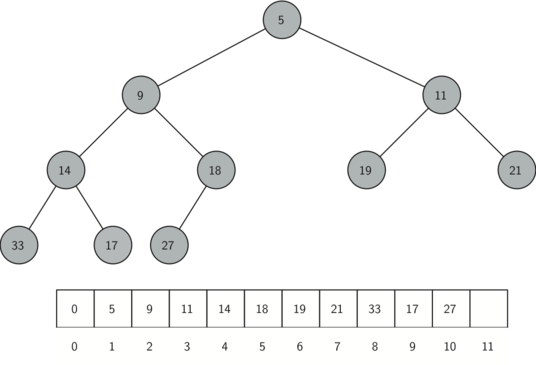Figure 2: A Complete Binary Tree, along with its Vector Representation¶

## 8.10.3. Heap Operations¶

We will begin our implementation of a binary heap with the constructor. Since the entire binary heap can be represented by a single vector, all the constructor will do is initialize the vector and an attribute currentSize to keep track of the current size of the heap. Listing 1 shows the C++ code for the constructor. You will notice that an empty binary heap has a single zero as the first element of heapList and that this zero is not used, but is there so that simple integer division can be used in later methods.

Listing 1

class BinHeap{
private:
vector<int> heapvector;
int currentSize;

public:
BinHeap(vector<int> heapvector){
this->heapvector = heapvector;
this->currentSize = 0;
}
}


The next method we will implement is insert. The easiest, and most efficient, way to add an item to a vector is to simply append the item to the end of the vector. The good news about appending is that it guarantees that we will maintain the complete tree property. The bad news about appending is that we will very likely violate the heap structure property. However, it is possible to write a method that will allow us to regain the heap structure property by comparing the newly added item with its parent. If the newly added item is less than its parent, then we can swap the item with its parent. Figure 2 shows the series of swaps needed to percolate the newly added item up to its proper position in the tree.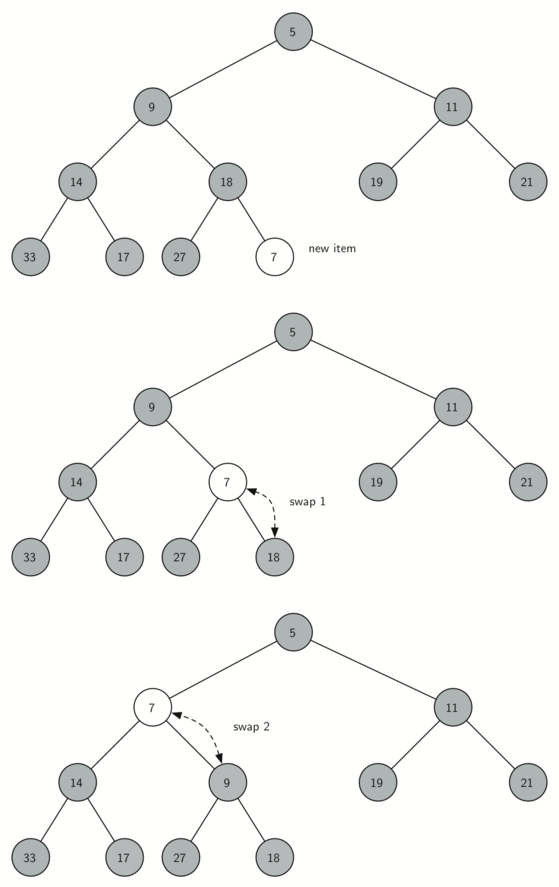Figure 2: Percolate the New Node up to Its Proper Position¶

Notice that when we percolate an item up, we are restoring the heap property between the newly added item and the parent. We are also preserving the heap property for any siblings. Of course, if the newly added item is very small, we may still need to swap it up another level. In fact, we may need to keep swapping until we get to the top of the tree. Listing 2 shows the percUp method, which percolates a new item as far up in the tree as it needs to go to maintain the heap property. Here is where our wasted element in heapvector is important. Notice that we can compute the parent of any node by using simple integer division. The parent of the current node can be computed by dividing the index of the current node by 2.

We are now ready to write the insert method (see Listing 3). Most of the work in the insert method is really done by percUp. Once a new item is appended to the tree, percUp takes over and positions the new item properly.

Listing 2

void percUp(int i){
while ((i / 2) > 0){
if (this->heapvector[i] < this->heapvector[i/2]){
int tmp = this->heapvector[i/2];
this->heapvector[i/2] = this->heapvector[i];
this->heapvector[i] = tmp;
}
i = i/2;
}
}


Listing 3

void insert(int k){
this->heapvector.push_back(k);
this->currentSize = this->currentSize + 1;
this->percUp(this->currentSize);
}


With the insert method properly defined, we can now look at the delMin method. Since the heap property requires that the root of the tree be the smallest item in the tree, finding the minimum item is easy. The hard part of delMin is restoring full compliance with the heap structure and heap order properties after the root has been removed. We can restore our heap in two steps. First, we will restore the root item by taking the last item in the vector and moving it to the root position. Moving the last item maintains our heap structure property. However, we have probably destroyed the heap order property of our binary heap. Second, we will restore the heap order property by pushing the new root node down the tree to its proper position. Figure 3 shows the series of swaps needed to move the new root node to its proper position in the heap.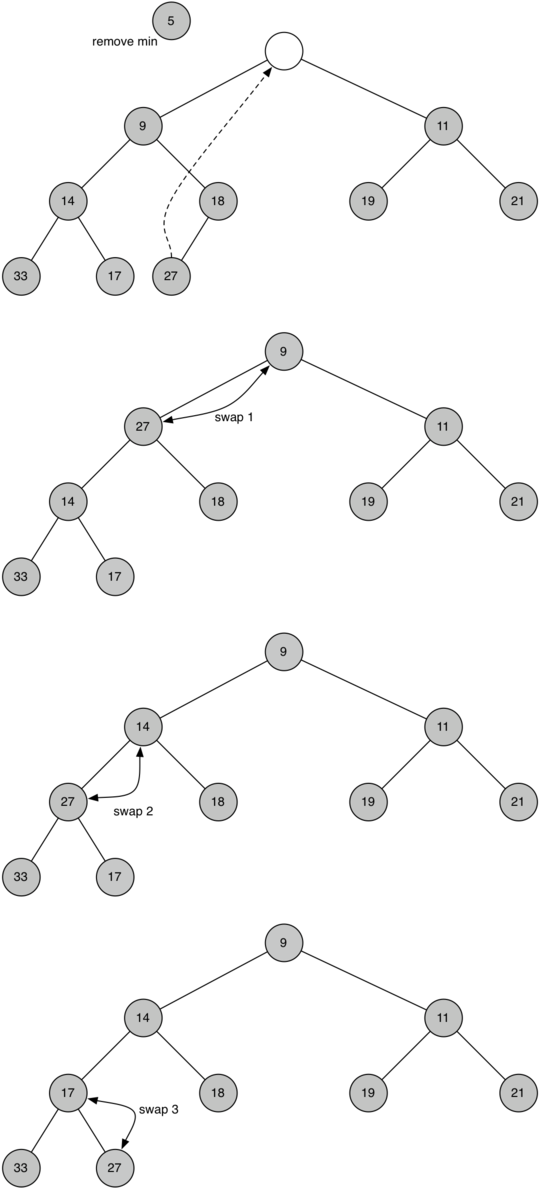Figure 3: Percolating the Root Node down the Tree¶

In order to maintain the heap order property, all we need to do is swap the root with its smallest child less than the root. After the initial swap, we may repeat the swapping process with a node and its children until the node is swapped into a position on the tree where it is already less than both children. The code for percolating a node down the tree is found in the percDown and minChild methods in Listing 4.

Listing 4

void percDown(int i){
while ((i*2) <= this->currentSize){
int mc = this->minChild(i);
if (this->heapvector[i] > this->heapvector[mc]){
int tmp = this->heapvector[i];
this->heapvector[i] = this->heapvector[mc];
this->heapvector[mc] = tmp;
}
i = mc;
}
}

int minChild(int i){
if (((i*2)+1) > this->currentSize){
return i * 2;
}
else{
if (this->heapList[i*2] < this->heapList[(i*2)+1]){
return i * 2;
}
else{
return (i * 2) + 1;
}
}
}


The code for the delmin operation is in Listing 5. Note that once again the hard work is handled by a helper function, in this case percDown.

Listing 5

int delMin(){
if (this->currentSize > 1){
int retval = this->heapvector;
this->heapvector = this->heapvector[this->currentSize];
this->currentSize = this->currentSize - 1;
this->heapvector.pop_back();
this->percDown(1);
return retval;
}
else{
int retval = this->heapvector;
this->heapvector = this->heapvector[this->currentSize];
this->currentSize = this->currentSize - 1;
this->heapvector.pop_back();
this->percDown(1);
return retval;
}
}


To finish our discussion of binary heaps, we will look at a method to build an entire heap from a vector of keys. The first method you might think of may be like the following. Given a vector of keys, you could easily build a heap by inserting each key one at a time. Since you are starting with a vector of one item, the vector is sorted and you could use binary search to find the right position to insert the next key at a cost of approximately $$O(\log{n})$$ operations. However, remember that inserting an item in the middle of the vector may require $$O(n)$$ operations to shift the rest of the vector over to make room for the new key. Therefore, to insert $$n$$ keys into the heap would require a total of $$O(n \log{n})$$ operations. However, if we start with an entire vector then we can build the whole heap in $$O(n)$$ operations. Listing 6 shows the code to build the entire heap.

Listing 6

void buildheap(vector<int> avector){
int i = avector.size() / 2;
this->currentSize = avector.size();
this->heapvector = avector;
while (i > 0){
this->percDown(i);
i = i - 1;
}
}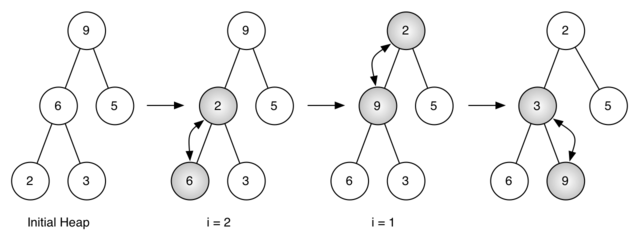Figure 4: Building a Heap from the vector [9, 6, 5, 2, 3]¶

Figure 4 shows the swaps that the buildHeap method makes as it moves the nodes in an initial tree of [9, 6, 5, 2, 3] into their proper positions. Although we start out in the middle of the tree and work our way back toward the root, the percDown method ensures that the largest child is always moved down the tree. Because the heap is a complete binary tree, any nodes past the halfway point will be leaves and therefore have no children. Notice that when i=1, we are percolating down from the root of the tree, so this may require multiple swaps. As you can see in the rightmost two trees of Figure 4, first the 9 is moved out of the root position, but after 9 is moved down one level in the tree, percDown ensures that we check the next set of children farther down in the tree to ensure that it is pushed as low as it can go. In this case it results in a second swap with 3. Now that 9 has been moved to the lowest level of the tree, no further swapping can be done. It is useful to compare the vector representation of this series of swaps as shown in Figure 4 with the tree representation.

i = 2  [0, 9, 5, 6, 2, 3]
i = 1  [0, 9, 2, 6, 5, 3]
i = 0  [0, 2, 3, 6, 5, 9]


The complete binary heap implementation can be seen in ActiveCode 1.

The assertion that we can build the heap in $$O(n)$$ may seem a bit mysterious at first, and a proof is beyond the scope of this book. However, the key to understanding that you can build the heap in $$O(n)$$ is to remember that the $$\log{n}$$ factor is derived from the height of the tree. For most of the work in buildHeap, the tree is shorter than $$\log{n}$$.

Using the fact that you can build a heap from a vector in $$O(n)$$ time, you will construct a sorting algorithm that uses a heap and sorts a vector in $$O(n\log{n}))$$ as an exercise at the end of this chapter.

You have attempted of activities on this page